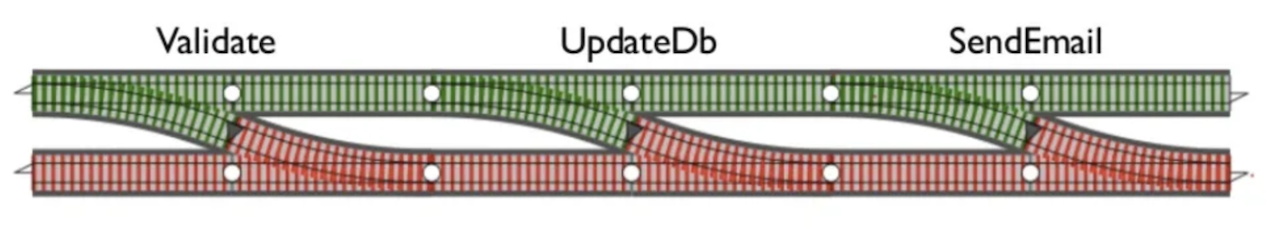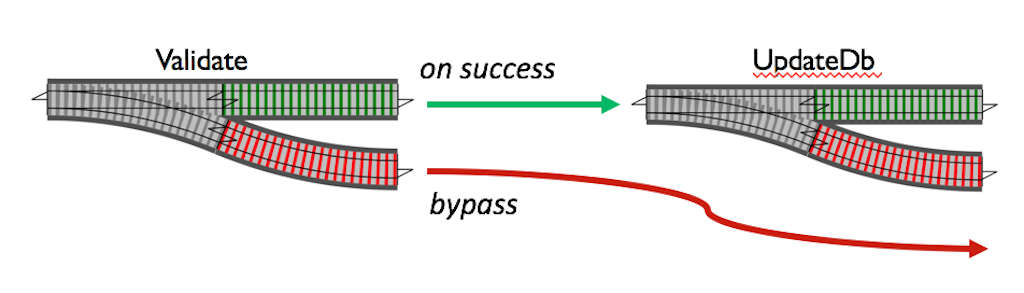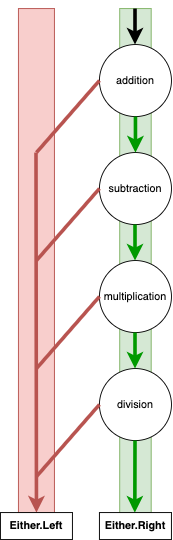# Error handling with Either in Java and Kotlin

## TLDR

``````  Either is either Left or Right with values inside.
Either is a union type.
It's a concept that is language agnostic.
It's right biased.
Left is used to transport/represent errors.
Right is used to transport/represent the successful data.
=flatMap= and =map= are used to chain them together.
bind is sugar over =flatMap= and =map= to make the code more sequential and easier to read.
It short circuits if it gets a Left.
Vavr for Java (no bind here).
Arrow for Kotlin (here you have bind).``````

## Introduction

Errors and exceptions in applications can be hard to handle and be aware of. Java tries to solve this with checked exceptions. Kotlin doesn't have checked exceptions so the compiler won't help or guide you.

One way of solving this is to represent errors and exceptions in a union type. There are a couple of different data types, one is Kotlin's built in Result. `Result` is a union type that can be of type A or Throwable. `Either` is a bit more general than `Result` and have `Left<A>` and `Right<B>`. `Left` and `Right` can contain any data type. By convention is `Left` used for error.

When a function returns a union type like `Either`. The compiler will make you aware of it and can guide you to handle both of the cases, `Left` and `Right`.

## Exceptions

Here is a small application that does 4 calculations. Each calculation is simulated to be executed on a different server, so the request could fail and throw an error.

This is pretty standard approach and often seen. In this case it's a small example so we can easily handle the exception. But if you forget the try/catch here you could end up in problem. Exceptions bring with them non-local jumps, which can make it hard to understand the flow of our code if you don't catch them locally.

``````  import java.io.IOException

// Simulates an external call to server
fun <A> runOnServer(f: () -> A): A =
if (Math.random() > 0.2) f()
else throw IOException("boom!")

fun addition(a: Int, b: Int): Int = runOnServer { a + b }
fun subtraction(a: Int, b: Int): Int = runOnServer { a - b }
fun multiplication(a: Int, b: Int): Int = runOnServer { a * b }
fun division(a: Int, b: Int): Int = runOnServer { a / b }

fun main(){
try {
val multiplicationResult = multiplication(subtractionResult, 10)
val divisionResult = division(multiplicationResult, 10)
println(divisionResult)
} catch (e: Exception) {
println("Error: \${e.message}")
}
}``````
``Error: boom!``

A function name could imply that it could throw an exception. For example `getPlayer(playerId)` hints that it will make I/O. But to be sure you need to look inside. This could be a disturbance and harms the purpose of an abstraction.

By only looking at the function names in this example it's easy to reason that only `division` could throw an `ArithmeticException`. But you need to look in the definitions to be sure.

If the functions in this example instead returned `Either<Error, Int>` it would be very clear that an error could occur.

## Railway programming

For this union types there are very general patterns and we can create simple functions that chain the functions together and short circuits if an error occurs. This is sometimes refereed to as railway programming.

In this railroad track you have 3 steps that needs to be executed in sequence. Each one of them can fail and if so it will short circuits and exit. So if Validate fails, UpdateDB and SendEmail won't be executed. But if Validate succeeds, it will go on to UpdateDb.We arrive at Validate. If we get an `Either.Right` we continue to UpdateDB. If we get an `Either.Left` we short circuit and exit.## flatMap, map and fold

There are 3 essential functions when working with railway programming and `Either`; `flatMap`, `map` and `fold`. Let's look at the implementations, they are super simple and you will see them or slight variations in different implementations of `Either`.

### flatMap

If we get an `Either.Right` we unpack the value and apply function `f` to it. If we get an `Either.Left` we should just pass it on. This is the short circuit mechanism. Just like in the railroad diagrams.

``````  public inline fun <A, B, C> Either<A, B>.flatMap(f: (B) -> Either<A, C>): Either<A, C> =
when (this) {
is Right -> f(this.value)
is Left -> this
}``````

### map

Is defined in terms of `flatMap` and only wraps the return from `flatMap` in an `Either.Right`.

``````  public inline fun <C> map(f: (B) -> C): Either<A, C> =
flatMap { Right(f(it)) }``````

### fold

If we get a `Either.Right`, run `ifRight` on its value, if we get an `Either.Left`, run `ifLeft` on its value. You don't need to end a chain with `fold`, there are a lot of other operators depending on the use case.

``````  public inline fun <C> fold(ifLeft: (A) -> C, ifRight: (B) -> C): C =
when (this) {
is Right -> ifRight(value)
is Left -> ifLeft(value)
}``````

## Either in Vavr

Let's put it all together.

We have the same 4 calculations, but we also have a `trap` function and an `Error` data class. Each calculation is surrounded in `trap`, so if `runOnServer` throws an exception the exception will be captured in the `Error` object together with a string and contained in a `Either.Left`. If everything is successful we get the calculated value in a `Either.Right`.

``````  import io.vavr.control.Either
import java.io.IOException

// A data class that holds data about the error
data class Error(val errorString: String, val exception: Exception)

// Simulates an operation that can go wrong
fun <A> runOnServer(f: () -> A): A =
if (Math.random() > 0.2) f()
else throw IOException("boom!")

// Wrap in Either.Left if exception, else in Either.Right
fun <A> trap(errorString: String, f: () -> A): Either<Error, A> =
try { Either.right(f()) }
catch (e: Exception) { Either.left(Error(errorString, e)) }

fun addition(a: Int, b: Int): Either<Error, Int> =
trap("Addition failed") { runOnServer { a + b } }
fun subtraction(a: Int, b: Int): Either<Error, Int> =
trap("Subtraction failed") { runOnServer { a - b } }
fun multiplication(a: Int, b: Int): Either<Error, Int> =
trap("Multiplication failed") { runOnServer { a * b } }
fun division(a: Int, b: Int): Either<Error, Int> =
trap("Division failed") { runOnServer { a / b } }

fun main() {
.flatMap { subtractionResult ->
multiplication(subtractionResult, 10)
.flatMap { multiplicationResult ->
division(multiplicationResult, 10)
.map { divisionResult ->
divisionResult
}
}
}
}
.fold(
{
// This is what we do if we get an Either.Left / Error
println(it)
},
{
// This is what we do if we get an Either.Right
println(it)
}
)
}``````
``Error(errorString=Division failed, exception=java.io.IOException: boom!)``

The code could be represented in a railway diagram. If `addition` returns a `Right<Int>` we continue to `subtraction` with the value inside `Right<Int>`, if `addition` fails it circuit breaks and returns a `Left<Error>` containing the error. It follow the same pattern all the way down. And returns a `Right<Int>` or a `Left<Error>`.## Either in Arrow

Vavr is aimed for Java and there is another implementation of Either aim for Kotlin in a lib called Arrow.

Here we replace the `Either` implementation from Vavr to Arrow. The code looks more or less the same. It's the same pattern with `flatMap`, `map` and `fold`.

``````  import arrow.core.Either
import arrow.core.flatMap
import java.io.IOException

// A data class that holds data about the error
data class Error(val errorString: String, val exception: Exception)

// Simulates an operation that can go wrong
fun <A> runOnServer(f: () -> A): A =
if (Math.random() > 0.2) f()
else throw IOException("boom!")

// Wrap in Either.Left if exception, else in Either.Right
fun <A> trap(errorString: String, f: () -> A): Either<Error, A> =
try { Either.Right(f()) } catch (e: Exception) { Either.Left(Error(errorString, e)) }

fun addition(a: Int, b: Int): Either<Error, Int> =
trap("Addition failed") { runOnServer { a + b } }
fun subtraction(a: Int, b: Int): Either<Error, Int> =
trap("Subtraction failed") { runOnServer { a - b } }
fun multiplication(a: Int, b: Int): Either<Error, Int> =
trap("Multiplication failed") { runOnServer { a * b } }
fun division(a: Int, b: Int): Either<Error, Int> =
trap("Division failed") { runOnServer { a / b } }

fun main() {
.flatMap { subtractionResult ->
multiplication(subtractionResult, 10)
.flatMap { multiplicationResult ->
division(multiplicationResult, 10)
.map { divisionResult ->
divisionResult
}
}
}
}
.fold(
{
// This is what we should do if we get an Either.Left / Error
println(it)
},
{
// This is what we do if everything when well
println(it)
}
)
}``````
``Error(errorString=Addition failed, exception=java.io.IOException: boom!)``

We still have the `flatMap`, `map` pyramid. Let's see if we can do something about it.

## Bind in Arrow

So let's look at how Arrow can fix this for us with something called `bind`. This gives us the exact same pattern as in the examples above, but it looks much more sequential and is much easier to read. The `either<Error,Int>` returns an `Either` and we fold over the result to take action on both the `Either.Left` and `Either.Right`.

``````import arrow.core.Either
import arrow.core.continuations.either
import kotlinx.coroutines.runBlocking
import java.io.IOException

// A data class that holds data about the error
data class Error(val errorString: String, val exception: Exception)

// Simulates an operation that can go wrong
fun <A> runOnServer(f: () -> A): A =
if (Math.random() > 0.2) f()
else throw IOException("boom!")

// Wrap in Either.Left if exception, else in Either.Right
fun <A> trap(errorString: String, f: () -> A): Either<Error, A> =
try { Either.Right(f()) } catch (e: Exception) { Either.Left(Error(errorString, e)) }

fun addition(a: Int, b: Int): Either<Error, Int> =
trap("Addition failed") { runOnServer { a + b } }
fun subtraction(a: Int, b: Int): Either<Error, Int> =
trap("Subtraction failed") { runOnServer { a - b } }
fun multiplication(a: Int, b: Int): Either<Error, Int> =
trap("Multiplication failed") { runOnServer { a * b } }
fun division(a: Int, b: Int): Either<Error, Int> =
trap("Division failed") { runOnServer { a / b } }

fun main() {
runBlocking {
either<Error,Int> {
val multiplicationResult = multiplication(subtractionResult, 10).bind()
val divisionResult = division(multiplicationResult, 10).bind()
divisionResult
}
}.fold(
{
// This is what we should do if we get an Either.Left / Error
println(it)
},
{
// This is what we do if everything when well
println(it)
}
)
}``````
``10``

The railway diagram for this code is the same as the one above.